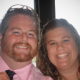## What is Your Favorite Number?

The WordPress Post-A-Week Challenge sends me a daily topic idea to consider for blog posts. Often, the prompts are not appropriate for a math jokes blog. For instance, some recent prompts have been:

• Grab the nearest book (or website) to you right now. Jump to paragraph 3, second sentence. Write it in a post.
• How do you find your muse?
• If you could bring one fictional character to life for a day, who would you choose?

But today’s prompt landed in my wheelhouse:

What is your favorite number, and why?

When Art Benjamin appeared on the Colbert Report, he said that 2,520 was his favorite number when he was a kid. When Stephen Colbert asked him why, he replied, “It was the smallest number that was divisible by all the numbers from 1 through 10.”

﻿Tonight, I asked my twin sons Alex and Eli what their favorite numbers are.

Eli: 5, 15, 55, because my favorite number is really 5, but 15 and 55 are triangular numbers that have 5’s in them.

Alex: 21, because my favorite numbers used to be 1 and 2, and because it’s the number of cards you deal when we play Uno (3 players, 7 cards each).

My favorite number is 153, for lots of reasons:

• ﻿﻿﻿It is the smallest non-trivial Armstrong (or narcissistic) number — that is, it is an n‑digit number that is equal to the sum of the nth powers of its digits: 13 + 53 + 33 = 153.
• Its prime factors are 3 and 17, and my birthday is 3/17.
• It is a triangular number. (Consequently, it’s the sum of 1 + 2 + 3 + … + 17.) As 351 is also a triangular number, 153 is also a reversible triangular number.
• It is the sum of the first five factorials: 1! + 2! + 3! + 4! + 5! = 153.
• The sum of its digits is 9, and the sum of its proper divisors is 92.
• It is one of only six known truncated triangular numbers, which means that 1, 15 and 153 are all triangular numbers.

﻿﻿﻿Mathematician John Baez claims that his favorite numbers are 5, 8, and 24.

Got a favorite number? Share it, as well as the reason it’s your favorite, in the comments.

Entry filed under: Uncategorized. Tags: , , , , , .

• 1.Jims  |  March 21, 2011 at 6:58 am

Zero
• It is the additive identity (n+0=n when ‘n’ is any number).
• 0 is not positive or negative.
• The only number in base 1 is 0.
• Like all Popular numbers, 0 has it’s own meme (Dividing by Zero causes amazing things in peoples imaginations). Note: the set of Popular numbers is undefined.
• 0 is a Whole number but not a Natural number, and that’s the only difference between them.
• 0 and the {} … need I say more?

I was going to have zero explanations, but that would’ve been pointless.

• 2.xander  |  March 21, 2011 at 11:21 am

I know that it is terribly cliché, but I rather like e (Euler’s number). It pops up all over the place—often in unexpected ways. Exponential functions behave nicely with respected to differentiation, and the exponential distribution is the only continuous memoryless distribution. e great.

• 3.Bon Crowder  |  March 21, 2011 at 1:37 pm

I like 4. Just simple. And square. And the first non-unit square whole number.

And nobody seems to have 4 as their fav. So it is less likely to be hogged by others.

• 4.Veky  |  March 22, 2011 at 2:47 am

Wow, and I thought I was unique in liking 4 and 153. 🙂

• 5.mixedmath  |  March 22, 2011 at 5:59 am

1001.

It’s the product of the first three ‘non-obvious’ primes, 7,11,13. These primes are the first whose divisibility rules take a little thought, and it turns out that 1001 provides the key as it’s only 1 away from 10^3.

• 6.zach  |  March 22, 2011 at 8:57 pm

my favorite number 705:

705 is the product of 3, 5, and 47 which are the unique prime numbers in the prime decomposition of the numbers 15 May 47 (5/15/47) my birthday.

The 705th Strong Number (Greek) is “To Number” “ἀριθμέω” (transliteration – “arithmeō”)

705 Is: The 4th 73-Gonal Number (72-gonal Numbers 1, 72, 213, 424, 705, 1038, 1477, 1968, 2529..)

705 Is: The 3rd 236-Gonal Number (236-gonal Numbers 1, 236, 705, 1408, 2345..)

705 Is: The First Lucas Pseudoprime

• 7.Bryan McDonald  |  March 28, 2011 at 10:33 am

“73 is the 21st prime number. Its mirror 37 is the 12th and its mirror 21 is the product of multiplying, hold on to your hats, 7 and 3. In binary, 73 is a palindrome, 1, 0, 0 1, 0, 0, 1 which backwards is 1, 0, 0 1, 0, 0, 1”

Sheldon Cooper
Big Bang Theory

• 8.Chloe  |  May 5, 2011 at 8:46 pm

36. The reason? It is the smallest number that is both a triangle and a square, and it’s square root is 6, the first mathematically perfect number. In fact, one could argue it is the most perfect, because it’s factors added AND multiplied both equal it. I am proud to say that I, a 14 year-old, figured this out on my own 😀